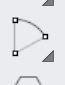# C# how to create a Arc(center, start, end)

Hello, I tried many things but I didn’t find the Arc (center, start, end) in c#. Where can I find or how to create it.yes not in Api but this method may be useful

``````  private void RunScript(Point3d start, Point3d center, Point3d end, Plane plane, ref object A, ref object B, ref object C, ref object D)
{

var   c = new Circle(plane, center, center.DistanceTo(start));
double t = 0;
var v1 = new Vector3d(start - center);
var _ = c.ClosestParameter(end, out t);
var p2 = c.PointAt(t);
var v2 = new Vector3d(p2 - center);
var ag1 = Vector3d.VectorAngle(Vector3d.XAxis, v1, plane);
var ag2 = Vector3d.VectorAngle(Vector3d.XAxis, v2, plane);
var arc = new Arc(c, new Interval(ag1, ag2));
A = arc;
B = new Line(center, start);
C = ag1;
D = ag2;
``````

Arc(start,center,end).gh (10.4 KB)

1 Like

That way of specifying an arc is underdefined. It works in Rhino as a command because Rhino uses the C-Plane in the active viewport to limit the problem from 3D to 2D. Also the motion of the mouse during the picking can be used to determine which segment of the arc you want (the clockwise, or the anti-clockwise one).

Grasshopper 2 does have a component close to this, the Arc Two Points component.

The first input is a plane rather than a point, and the outputs are both the clockwise and the anti-clockwise arcs that satify the input. You’ll then need to decide which of those two is the one you really wanted.

2 Likes

Many thanks for your script, it helps me so much to create my LandXml (Trasse) reader for railways.

Nice to see that Grasshopper is getting new nodes

1 Like